Courses

# Notes | EduRev

## Class 10 : Notes | EduRev

The document Notes | EduRev is a part of the Class 10 Course Class 10 Mathematics by VP Classes.
All you need of Class 10 at this link: Class 10

Ques 1: Which term of the AP: 121, 117, 113, ..., is its first negative term?
[Hint: Find n for an < 0]
Sol:
We have the A.P. having a = 121 and d = 117 - 121 = - 4
∴  an = a + (n - 1) d
= 121 + (n - 1) × (- 4)
= 121 - 4n + 4
= 125 - 4n
For the first negative term, we have
an < 0
⇒ (125 - 4n) < 0
⇒ 125 < 4n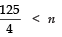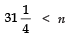or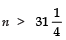Thus, the first negative term is 32nd term.

Ques 2: The sum of the third and the seventh terms of an AP is 6 and their product is 8. Find the sum of first sixteen terms of the AP.
Sol: Here, T3 + T7 = 6  and  T3 × T7 = 8
Let the first term = a and the common difference = d
∴ T3 = a + 2d  and  T7 = a + 6d
∵ T3 + T7 = 6
∴ (a + 2d) + (a + 6d) = 6
⇒ 2a + 8d = 6
⇒ a + 4d = 3 ...(1)
Again T3 × T7 = 8
∴ (a + 2d) × (a + 6d) = 8
⇒ (a + 4d - 2d) × (a + 4d + 2d) = 8
⇒ [(a + 4d) - 2d] × [(a + 4d) + 2d] = 8
⇒ [(3) - 2d] × [(3) + 2d] = 8 [From (1)]
⇒ 32 - (2d)2 = 8
⇒ 9 - 4d2 = 8
⇒ - 4d2 = 8 - 9 = - 1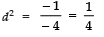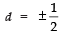When d = 1/2.
From (1), we have: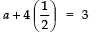⇒ a + 2 = 3 or a = 3 − 2 = 1
Now, Using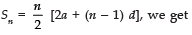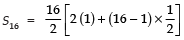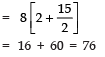i.e., the sum of first 16 terms = 76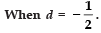From (1), we have: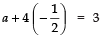⇒ a − 2 = 3 ⇒ a = 5
Again, the sum of first sixteen terms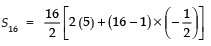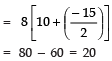i.e., the sum of first 16 terms = 20

Ques 3: A ladder has rungs 25 cm apart (see figure). The rungs decrease uniformly in length from 45 cm at the bottom to 25 cm at the top. If the top and the bottom rungs are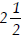m apart, what is the length of the wood required for the rungs?
[Hint: Number of rungs =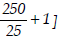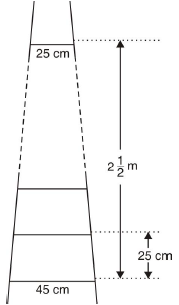Sol: Total distance between bottom to top rungs
=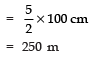Distance between two consecutive rungs = 25 cm
∴ Number of rungs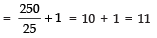Length of the 1st rung (bottom rung) = 45 cm
Length of the 11th rung (top rung) = 25 cm
Let the length of each successive rung decrease by x cm
∴ Total length of the rungs
= 45 cm + (45 - x) cm + (45 - 2x) cm + ..... + 25 cm
Here, the numbers 45, (45 - x), (45 - 2x), ....., 25 are in an A.P. such that
First term ‘a’ = 45
Last term ‘l’ = 25
Number of terms ‘n’ = 11
∴ Using,
Sn = n/2 [a + l], we have
S11 = 11/2 [45 + 25]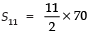⇒ S11 = 11 × 35 = 385
∴ Total length of 11 rungs = 385 cm
i.e., Length of wood required for the rungs is 385 cm.

Ques 4: The houses of a row are numbered consecutively from 1 to 49. Show that there is a value of x such that the sum of the numbers of the houses preceding the house numbered x is equal to the sum of the numbers of the houses following it. Find this value of x.
[Hint : Sx – 1 = S49 – Sx]
Sol: We have, the following consecutive numbers on the houses of a row;
1, 2, 3, 4, 5, ....., 49.
These numbers are in an A.P., such that
a = 1
d = 2 - 1 = 1
n = 49
Let one of the houses be numbered as x
∴ Number of houses preceding it = x - 1
Number of houses following it = 49 - x
Now, the sum of the house-numbers preceding x is given by:
Using Sn = n/2 [2a + (n - 1) d]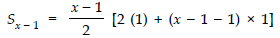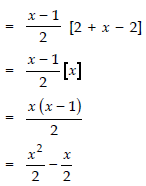The houses beyond x are numbered as
(x + 1), (x + 2), (x + 3), ....., 49
∴ For these house numbers (which are in an A.P.),
First term (a) = x + 1
Last term (l) = 49
∴ Using Sn = n/2 [a + l], we have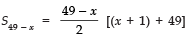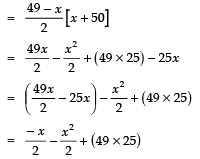According to the question,
[Sum of house numbers preceding x] = [Sum of house numbers following x]
i.e.,  Sx − 1 = S49 − x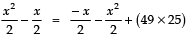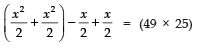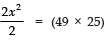⇒ x2 = (49 × 25)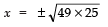⇒ x = ± (7 × 5) = ± 35

But x cannot be taken as -ve
∴ x = 35

Ques 5: A small terrace at a football ground comprises of 15 steps each of which is 50 m long and built of solid concrete.
Each step has a rise of 1/4m  and a tread of 1/2m. (see Fig.). Calculate the total volume of concrete required to build the terrace.
[Hint: Volume of concrete required to build the first step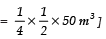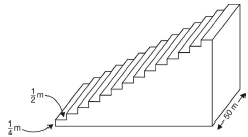Sol: For 1st step:
Length = 50 m, Breadth = 1/2m, Height = 1/4m
∴ Volume of concrete required to build the 1st step
= Volume of the cuboidal step
= Length × Breadth × height
=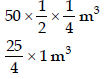For 2nd step:
Length = 50 m, Breadth = 1/2m, Height =∴ Volume of concrete required to build the 2nd step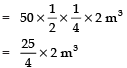For 3rd step:
Length = 50 m, Breadth = 1/2m, Height =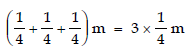∴ Volume of concrete required to build the 3rd step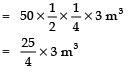...........  ...........  ...........  ...........  ...........
Thus, the volumes (in m3) of concrete required to build the various steps are: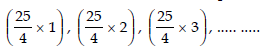obviously, these numbers form an A.P. such that
a = 25/4
d =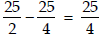Here, total number of steps n = 15
Total volume of concrete required to build 15 steps is given by the sum of their individual volumes.
∴ Using,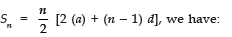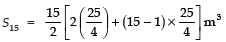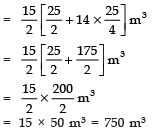Thus, the required volume of concrete is 750 m3.

Offer running on EduRev: Apply code STAYHOME200 to get INR 200 off on our premium plan EduRev Infinity!

132 docs

,

,

,

,

,

,

,

,

,

,

,

,

,

,

,

,

,

,

,

,

,

;